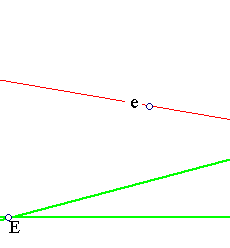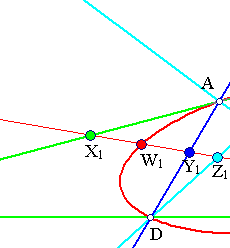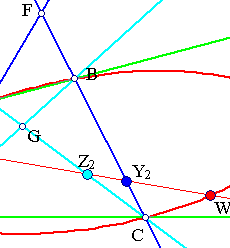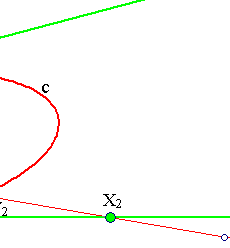##Desargues involution theorem

This extends the basic figure by which we define involutive homographies on lines (see InvolutionBasic.html ). This is done by intersecting a line (e) with the conics (c) passing through four points {A,B,C,D} in general position (each tripple non-collinear). Also we restrict the line to not pass through the four basic points {A,B,C,D} as well as not pass through the intersection points of the opposite sides {E,F,G} of the quadrilateral.
The theorem of Desargues asserts that there is an involution f on (e) which interchanges every pair of points (W1,W2) intercepted on (e) by the conics passing through the four points {A,B,C,D}.The proof is essentially the same as the one given in the above reference. We consider the bundles of lines A(W1,D,C,W2) and B(W1,D,C,W2). They define the same cross ratio through the intercepts on line (e): (W1,Y1,Z2,W2) = (W1,Z1,Y2,W2) = (W2,Y2,Z1,W1). Thus there is a homographic relation f on line (e) mapping: f(W1)=W2, f(Y1)=Y2, f(Z1)=Z2 and f(W2)=W1. Since it interchanges W1 and W2 it is involutive. As such it is uniquely defined by the requirement to map Z1 to Z2 and Y1 to Y2. Thus it is independent of the conic (c).

A special case arises when D tends to coincide with A. In that case DA becomes a tangent to the conic and the theorem of Desargues beomes a property of triangles inscribed in conics. This is discussed in DesarguesInvolution2.html .
The theorem of Desargues transfers with the same wording to conics defined in the complex projective space. There every two conics have four intersection points and the arguments transfer verbatim. A consequence of the validity of the theorem in the complex case is demonstrated in the file DesarguesInvolutionComplex.html .

### See Also

CrossRatio0.html
DesarguesInvolution2.html
DesarguesInvolution3.html
DesarguesInvolutionComplex.html
FourPtsAndTangent.html
FourPtsAndTangent2.html
HomographicRelation.html
HomographicRelationExample.html
InvolutionBasic.html

### References

Doerrie, Heinrich 100 Great Problems of Elementary Mathematics New York, Dover, 1965, p. 269.

 Produced with EucliDraw© http://users.math.uoc.gr/~pamfilos/